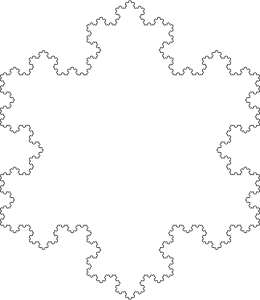#Koch Curve

Math Lair Home > Topics > Koch CurveThe Koch curve (also known as the von Koch curve) is a type of fractal. It was first described in a 1904 paper by Swedish mathematician Helge von Koch, making it one of the first fractal curves to be described.

It's pretty simple to create:

• Break the line into three equal line segments.
• Using the middle line segment, create an equilateral triangle with that segment as the base, and then delete the original line segment.
• Repeat the above process indefinitely.A Koch snowflake.

Repeating the process an infinite amount of times creates the Koch curve. The first seven iterations of this process are illustrated at the top of the page.

A similar curve is the Koch snowflake, which is the same as a Koch curve, except the starting point is a triangle, which results in the shape on the right, one that looks a little bit like a snowflake. Even though the length of the curve is infinite, the area enclosed by the curve is finite; it converges on an area 8/5 of the area of the original triangle.

Here are some activities to try:

• If you have experience with infinite series, prove that the area of the Koch snowflake is 8/5 of the area of the original triangle.
• Try constructing an anti-snowflake by drawing the triangles inward instead of outward. What is the limiting area of this curve?
• What would happen if you were to construct the curve in a different manner, such as by using other polygons instead of triangles, or by constructing multiple polygons on each side?

Sources used (see bibliography page for titles corresponding to numbers): 17, 56.